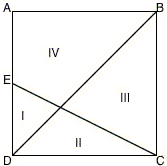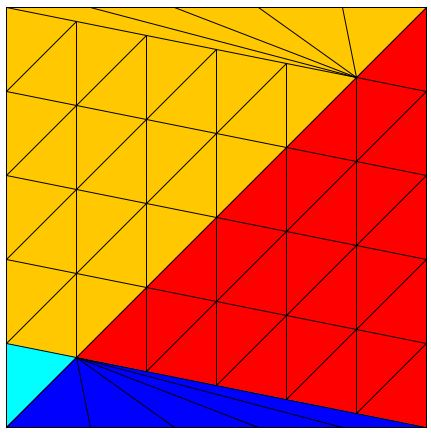# Areas in Square by Dissection

The July 15 issue of the NCTM Newsletter contained the following problem:

In the figure below, quadrilateral ABCD is a square, and E is the midpoint of the side AD. How do the areas of regions I, II, III, and IV compare? Another way to think about this is to consider the question, What are the ratios of the areas of the four subdivisions, I : II : III : IV?The August issue offered a very nice solution by dissection.

The applet below presents a generalization of the problem and its solution.

### If you are reading this, your browser is not set to run Java applets. Try IE11 or Safari and declare the site https:///www.cut-the-knot.org as trusted in the Java setup.What if applet does not run?

Let DE = AD/N, N ≥ 2. Then

I : II : III : IV = 1 : N : N² : N² + N - 1.

The key to these ratios is the fact that, if points are marked on the sides of a triangle that divide the sides into N parts then the lines parallel to the sides joining the points split the triangle into N² equal triangles. This follows from the simple dependency of the area on the linear dimensions of a triangle (but is true for any other shape.) If the sides of a triangle are increased by the factor of N, then its area increases by the factor of N². This is the same dependency that was used by Euclid in his second and less known proof of the Pythagorean theorem.